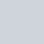# Statistical Hypothesis Testing step by step procedure

•
•
•
•
•
•
•
•
•

Statistical hypothesis testing is a procedure of a test on the basis of observed data modelled as the realised values taken by a collection. According to Investopedia,

“Hypothesis testing is an act in statistics whereby an analyst tests an assumption regarding a population parameter.”

## What is hypothesis testing?

In statistics, we may divide statistical inference into two major part: one is estimation and another is hypothesis testing. Before hypothesis testing we must know about hypothesis. so we can define hypothesi as below-

A statistical hypothesis is a statement about a population which we want to verify on the basis of information which contained in a sample.

### Example of statistical hypothesis testing

Few examples of statistical hypothesis related to our daily life are given below-

• The court assumes that the indicted person is innocent.
• A teacher assumes that 80% of the student of his college is from a lower-middle-class family.
• A doctor assumes that 3D(Diet, Dose, Discipline) is 95% effective to the diabetes patient.
• A beverage company claims that its new cold drinks are superior to the other drinks available in the market, etc.

A statistical test mainly involves four steps:

• Evolving a test statistic
• To know the sampling distribution of the test statistic
• Selling of hypotheses testing conventions
• Establishing a decision rule that leads to an inductive inference about the probable truth.

### Types of statistical hypothesis

• Null hypothesis
• Alternative hypothesis

### Null hypothesis

A null hypothesis is a statement, which tells us that no difference exists between the parameter and the statistic being compared to it. According to Fisher, any hypothesis tested for its possible rejection is called a null hypothesis and is denoted by H0.

### Alternative hypothesis

The alternative hypothesis is the logical opposite of the null hypothesis. The rejection of the null hypothesis leads to the acceptance of the alternative hypothesis. It is denoted by H1.

For example, with a coin-tossing experiment, the null and alternative hypothesis may be formed as,

H0: the coin is unbiased.

H1: the coin is biased.

Depending on the population distribution, the statistical hypothesis are two types,

• Simple hypothesis: when a hypothesis completely specifies the distribution of the population, then the hypothesis is called a simple hypothesis.
• Composite hypothesis: when a hypothesis does not completely specify the distribution of the population, then the hypothesis is called a composite hypothesis.

### One thought on “Statistical Hypothesis Testing step by step procedure”

1.Camryn

can hemp be used as animal feed